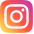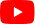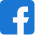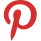# Leon and Turtle Aquarium - Math riddle

###### 8.Math Riddles
`Leon works at the aquarium. When he tries to put each turtle in its own tank, he has one turtle too many. But if he puts two turtles per tank, he has one tank too many. How many turtles and how many tanks does Leon have? `

```
```

• A.1,4
• B.2,1
• C.3,4
• D.4,2
C.3,4
• ```Explanation :

1 turtle in each tank = 1 turtle too many

2 turtles in each tank = 1 tank too many

Using one of the better known heuristic to solve this question

Before that, we know that:

2 turtles in each tank = 1 tank too many

2 turtles in each tank = 2 turtles short

First, find the difference in the number of turtles in the two scenarios:

First scenario = 1 turtle too many

Second scenario = 2 turtles short

Difference in turtles = 1 - (- 2)

Difference in turtles =  3

Second, find the difference in the distribution of turtles:

First scenario = 1 turtle each tank

Second scenario = 2 turtles each tank

Difference in turtle = 2 - 1

Difference in turtles = 1

Find the number of tanks:

Number of tanks = 3 ÷ 1

Number of tanks = 3

Find the number of turtles:

Using the first scenario, there was one turtle too many

Number of tanks = 3

Number of turtles = 3 + 1

Number of turtles = 4

There are 4 turtles and 3 tanks.```
•Mindyourlogic
• Follow

•Mindyourlogic Hindi
• Follow

•Mindyourlogic English
• Follow

•Mindyourlogic Hindi
• Follow

•Mindyourlogic
• Follow